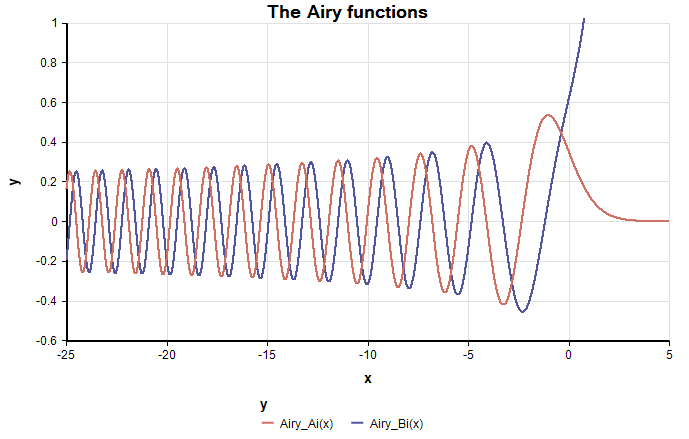# Airy functions

(Redirected from Airy Ai deriv)

(New to Analytica 6.0)

Airy functions are advanced mathematical functions that are typically denoted as $\displaystyle{ Ai(x) }$ and $\displaystyle{ Bi(x) }$ in scholarly articles. The are used in many fields including quantum mechanics, electrodynamics, electromagnetism, analysis of combinatory complexity, computation of orthogonal polynomials and spectra, optics, combinatorics, solid state physics, and the study of radiation.

The Airy functions are the two linearly independent solutions to the differential equation:

$\displaystyle{ y'' = x y }$

## Functions

Airy_Ai(x): The Airy function of the first kind.
Airy_Bi(x): The Airy function of the second kind.
Airy_Ai_deriv(x): The first derivative of Airy_Ai(x)
Airy_Bi_deriv(x): The first derivative of Airy_Bi(x)
Airy_Ai_zero(nth): The «nth» zero, or root, of Airy_Ai(x)
Airy_Bi_zero(nth): The «nth» zero, or root, of Airy_Bi(x)

## Examples

Index x := Sequence(-25,5,1m)
[ Airy_Ai(x), Airy_Bi(x) ]Variable n := 1..25
[Airy_Ai_zero(n),Airy_Bi_zero(n) ]